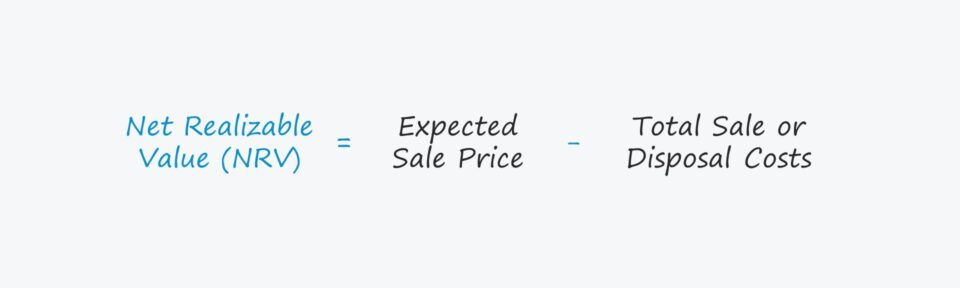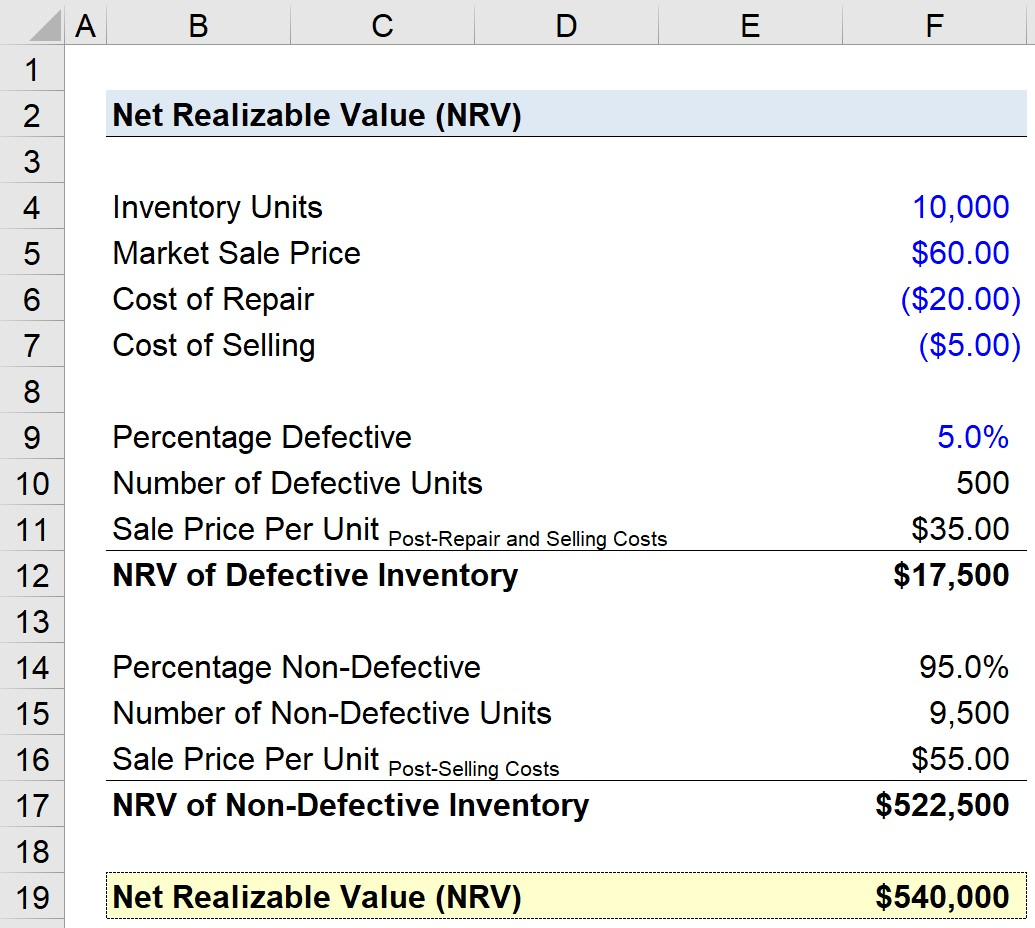Welcome to Wall Street Prep! Use code at checkout for 15% off.# Net Realizable Value (NRV)

Guide to Understanding the Net Realizable Value (NRV)## How to Calculate Net Realizable Value (NRV)

The net realizable value (NRV) is an accounting method to appraise the value of an asset, namely inventory and accounts receivable (A/R).

In accordance with the principle of conservatism, the value of assets must be recorded on a historical basis per U.S. GAAP accounting standards to impede companies from inflating the carrying value of their assets.

For example, a publicly-traded company must recognize the value of its inventory on the balance sheet at either the historical cost or the market value, based on whichever option is lower.

In effect, companies are prevented from overstating the value of their inventory, which reduces the risk of misleading investors.

Therefore, the net realizable value (NRV) estimates the amount that a seller would expect to receive if the asset in question was sold, net of any selling or disposal costs.

The accounting standards established under U.S. GAAP require companies to strictly abide by the conservatism principle to appraise the value of assets.

The steps to calculate the net realizable value (NRV) are as follows:

1. Determine the Expected Sale Price, i.e. the Fair Market Value (FMV)
2. Calculate the Total Costs Associated with the Asset Sale, i.e. Marketing, Advertising, Delivery
3. Subtract the Sale or Disposal Costs from the Expected Sale Price

## Net Realizable Value Formula (NRV)

The formula for calculating net realizable value (NRV) is the difference between the expected sale price and the total sale or disposal costs.

Net Realizable Value (NRV) = Expected Sale PriceTotal Sale or Disposal Costs
• Expected Sale Price → The estimated price at which the asset could be sold in the open markets.
• Total Sale or Disposal Costs → The costs expected to be incurred in the process of selling the asset.

## NRV Calculation Example

For example, suppose a company’s inventory was purchased for \$100.00 per unit two years ago, but the market value is now \$120.00 per unit at present.

Q. If the associated costs with the sale of the inventory is \$40.00, what is the net realizable value?

After subtracting the selling costs (\$40.00) from the market value (\$120.00), the NRV of the company’s inventory is \$80.00.

• Net Realizable Value (NRV) = \$120.00 – \$40.00 = \$80.00

On the accounting ledger, an inventory impairment of \$20.00 would then be recorded.

## Net Realizable Value Calculator (NRV)

We’ll now move to a modeling exercise, which you can access by filling out the form below.Submitting...

## 1. Inventory Accounting Assumptions

Suppose a manufacturing company has 10,000 units of inventory that it intends to sell.

The market value on a per-unit basis is \$60.00, and the associated selling costs are \$5.00 per unit, but 5.0% of the inventory is defective and requires repairs, which costs \$20.00 per unit.

• Inventory Units = 10,000 Units
• Market Sale Price = \$60.00
• Cost of Selling = \$5.00
• Cost of Repair = \$20.00

Since 5% of the inventory is defective, that means 500 units require repairs.

• % Defective Inventory = 5.0%
• Defective Units = 500 Units

The sale price per unit for the defective units – upon incurring the repair and selling costs – is \$35.00 per unit.

• Sale Price Per Unit (Defective) = \$60.00 – \$20.00 – \$5.00 = \$35.00

## 2. NRV Calculation Example

The NRV of the defective Inventory is the product of the number of defective units and the sale price per unit after the repair and selling costs.

• Net Realizable Value (NRV)= 500 Units × \$35.00 = \$17,500

The percentage of non-defective inventory units is 95%, so there are 9,500 non-defective units.

• % Non-Defective Inventory = 95.0%
• Non-Defective Units = 9,500 Units

To calculate the sale price per unit for the non-defective units, only the selling costs need to be deducted, which comes out to \$55.00.

• Sale Price Per Unit (Non-Defective) = \$55.00

We’ll multiply the number of non-defective units by the sale price per unit after selling costs, resulting in the NRV of non-defective inventory of \$522,500

The net realizable value (NRV) of our hypothetical company’s inventory can be calculated by adding the defective NRV and the non-defective NRV, which is \$540,000.

• Net Realizable Value (NRV) = \$17,500 + \$522,500 = \$540,000Step-by-Step Online Course

### Everything You Need To Master Financial Modeling

Enroll in The Premium Package: Learn Financial Statement Modeling, DCF, M&A, LBO and Comps. The same training program used at top investment banks.

Inline Feedbacks